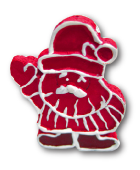### Challenge from 21. December

Everything Must Go!

Authors: , Max Klimm

Challenge:

After Christmas, Santa Claus tries to get rid of all the presents that have been rejected or were returned. To this end, he auctions off whatever is still there. The only currency accepted at the North Pole are fir cones, which can be divided arbitrarily. Santa Claus always uses second price auctions with reserve price. In such an auction every bidder hands in a sealed bid. The highest bid wins as long as it is at least as high as the reserve price. In this case, the profit is equal to the maximum of the second highest bid and the reserve price. The profit is kept by Santa Claus for buying presents the next Christmas season.

1. The first article considered for auction is a tacky vase in which only Cato shows interest. From previous years' experience, it is known that Cato will bid either one or two cones, each with probability 1/2.

2. The second article to be auctioned off is a sock sorting device. Only Luca is willing to bid for it. Luca will bid either one, two or three fir cones, each with probability 1/3.

3. The third article in the auction is a solar powered paperweight. Elisa and Frana will hand in bids for this item. Elisa bids either one or three fir cones, each with a probability of 1/2, and Frana bids either two or four fir cones, each with a probability of 1/2. Both elves bid independently of each other.

4. The fourth article that is called up is a pottery flower bouquet in which only Cato is interested. Cato will either bid one or two cones, each with probability 1/2. The bid and the interest of Cato in the flower bouquet is independent from the first auction.

Now, Santa contemplates on how to choose the reserve price for each auction in order to maximise the expected profit. The expected profit of an auction with reserve price is the sum of all possible profits, each multiplied with the probability of obtaining this profit. Santa wrote down the following conjectures concerning the above auctions:

1. For the first auction, a reserve price of 2 gives an expected profit of 1.

2. For the first auction, a reserve price of 2 is optimal, i. e., there is no other reserve price that yields a strictly higher expected profit.

3. For the third auction, a reserve price of 4 is optimal.

4. The expected profit of the second auction with optimal reserve price is smaller than the expected profit in the third auction with the reserve price of 0.

5. Instead of selling the vase and the bouquet in two separate auctions, one may also sell them together as bundle. Assuming that Cato will bid for the bundle the sum of the bids for the items within, a reserve price of 3 for the bundle gives an expected profit of 9/4.

6. The reserve price of 3 for the bundle is optimal.

7. Assume now that Cato will bid one or four cones in the first and fourth auction, each with probability 1/2. Then, selling both items in a bundle gives more profit than selling both items separately (where we investigate the expected profit corresponding to the optimal reserve price for each auction).

Which of the statements (A) to (G) are correct?

Artwork: Friederike Hofmann1. None of the statements (A) to (G) is correct.

2. Only statements (A) and (B) are correct.

3. Only statements (A), (B), and (C) are correct.

4. Only statements (A), (B), and (D) are correct.

5. Only statements (A), (B), (D), and (E) are correct.

6. Only statements (A), (B), (C), (D), and (E) are correct.

7. Only statements (A), (B), (D), (E), and (F) are correct.

8. Only statements (A), (B), (E), (F), and (G) are correct.

9. Only statements (A), (B), (D), (E), (F) and (G) are correct.

10. All of the statements (A) to (G) are correct.